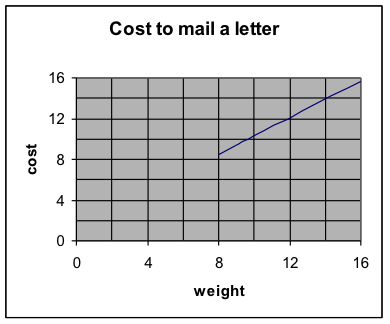## H1.04: Example 3

Example 3: For a certain type of letter sent by Federal Express, the charge is $8.50 for the first 8 ounces and$0.90 for each additional ounce (up to 16 ounces.) How much will it cost to send a 12-ounce letter?

Solution:

1. What are the two variables and their units?

Ans. Weight and cost. Weight is measured in ounces and cost is measured in dollars.

2. What will we predict? (Make it y.)  What are some points?

Ans. We will predict cost. So y = cost. Then x = ounces and x must be greater than or equal to 8. Cost must be at least $8.50. Eight ounces cost 8.50. So x = 8 and y = 8.50. Use (8, 8.50) Nine ounces cost 9.40. So x = 9 and y = 9.40. Use (9, 9.40) 3. Is a linear model appropriate? Yes, because the cost increases by the same amount for each additional ounce. 4. Slope Find the slope: m = rise / run Let (8, 8.50) be the first point and (9, 9.40) be the second point. $m=\frac{{{y}_{2}}-{{y}_{1}}}{{{x}_{2}}-{{x}_{1}}}=\frac{9.40-8.50}{9-8}=\frac{0.90}{1}=0.90$ Interpret the slope, using the units of the numbers in the problem: (As x increases by 1, y increases by m.) For each additional ounce, the cost increases by$0.90.

(Notice that was given in the problem. So really we didn’t need to compute the slope. If we had recognized the interpretation of it, we could have just picked it out of the statement of the problem and skipped step 8.)

5. Write the formula for the linear relationship:

(Choose either point and use the point-slope form of the line. Then simplify it to the slope-intercept form of the line.)

Choose (8, 8.50) for the point and use the slope of 0.90.

\begin{align}&y-{{y}_{0}}=m(x-{{x}_{0}})\\&y-8.50=0.90(x-8)\\&y-8.50=0.90x-7.20\\&y=0.90x+1.30\\\end{align}

6. Interpret the y-intercept.

(The value of y is b when $x=0$.)

When the envelope weighs 0 ounces, the model says that the cost is 1.30. Since the model is stated to only be good for weights of at least 8 ounces, then this is not a useful observation. 7. Use the equation to make the requested prediction. Write the result in a sentence, with units. For 12 ounces, x = 12: \begin{align}&y=0.90x+1.30\\&y=0.90(12)+1.30\\&y=10.80+1.30\\&y=12.10\\\end{align} So a 12-ounce letter will cost12.10.

8. Sketch a graph from the original problem information, use it to estimate the answers to the prediction questions, and determine whether your answers from the algebraic formula are reasonable.

 weight cost 8 8.50 9 9.40

By hand, plot these points, draw the line, extend it to the limits for the variables, and use it to estimate the answer to the question.Using the graph, the cost for a letter of 12 ounces is a little more than \$12.

That is consistent with the result from using the linear formula.

The prediction is reasonable.

Notice that the graph here only includes a line for the parts where the linear model is appropriate. In other words, it doesn’t extend below a weight of 8 ounces. This helps illustrate why the y-intercept here is not very useful.